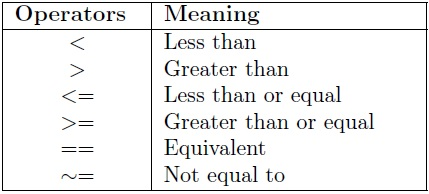If the expression is true, then the commands are executed, otherwise the program continues with the next command immediately beyond the end statement.
The simplest form of if  statements is

if
statement1 ;
end

If the condition is true, the statement1 is executed, but if the condition is false, nothing happens. We use relational operators to create condition in if statement. Several relational operators have been shown in the following table :Suppose that an expression is A < B. When this condition is true, then block of statements will be executed. When this condition is false, then block of statements will not be executed.
The syntax of an if…else statement in MATLAB is

if condition
statementA ;
else
statementB ;
end

If the condition is true, then the if block of code will be executed, otherwise else block of code will be executed.

The for loop is a loop that executes a block of statements a specifi ed number of times. The syntax of for loop has the form:

for index = values
statement1 ;
statement2 ;
. . .
end

The loop begins with the for statement and ends with the end statement.
Example
Write MATLAB script to compute and display 10!.

function f= factorial ( a )
f =1;
for n=2:a
f=f ∗n ;
end

Example
Write MATLAB function to display the most negative element in a given matrix

function mn=mostnegative (A)
%input matrixA
%find most negative element in matrix A
[m, n]= size (A) ;
mn=0;
for I=1:m
for J=1:n
if A(I,J)&lt;= mn mn=A(I,J) ;
end
end
end

A=[-1 -4 -3; -5 -2 -6; -7 -9 -8 ]

>> mn = mostnegative(A)
mn =
-9

The while loop is a loop that executes a block of statements repeatedly as long as the expression is true. The syntax is :

while expression
statement1 ;
statement2 ;
. . .
end

The for and while loops can be terminated using the break command.

Example
Write a MATLAB function to display even numbers in vector.

function E = even (B)
% input : vector B
% output : vector E
% This function displays even number
[~,n]= size (B) ;
j=1;
c=1;
while j&lt;=n
if mod(B(1,j),2)==0
E(1,c)=B(1,j) ;
c=c+1;
end
j=j +1;
end